# Explaining the Monty Hall Problem, One Of Math's Most Perplexing PuzzlesPublished10Comments1.3kShares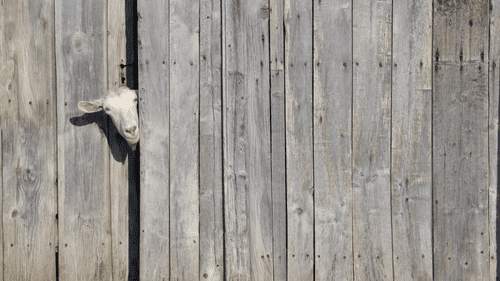Behind this door might be the car of your drea— oh, no, it's a goat. Image credit: Petar Paunchev/Shutterstock.com

Imagine you’re on a gameshow, and you’re given the choice of three doors. Behind one door is a brand new car; behind the other two, goats. The game is simply to pick a door: whatever is behind it, you win.

Now, goats are lovely animals, but you really want that car, so you decide to plump for door number one.

“Would it change your mind,” the host says mischievously, “if I told you the car isn’t behind door three?”

Well – would it?

This thought experiment is called the Monty Hall Problem, and it’s caused quite a bit of controversy in the math world over the years. It was originally published as a letter to the editor in a 1975 edition of the American Statistician, but it really took off in 1990, when Parade writer Marilyn vos Savant was posed it by a reader for her “Ask Marilyn” column.

Have you decided whether you should switch to door two yet? If you want the best chance of winning that car, you should.

Confused? You’re in good company. When vos Savant gave that answer in her column, tens of thousands of readers – nearly 1,000 PhDs among them – wrote in to complain that she was wrong. Paul Erd?s, a mathematician so prolific and respected that his name has become a benchmark for collaboration, refused to accept the result for years (eventually a friend sat him in front of 100,000 computer simulations that proved the result without a doubt, and he was forced to accept the conclusion, but he wasn’t happy about it.)

But it’s true: swap doors, and you double your chance of winning the car.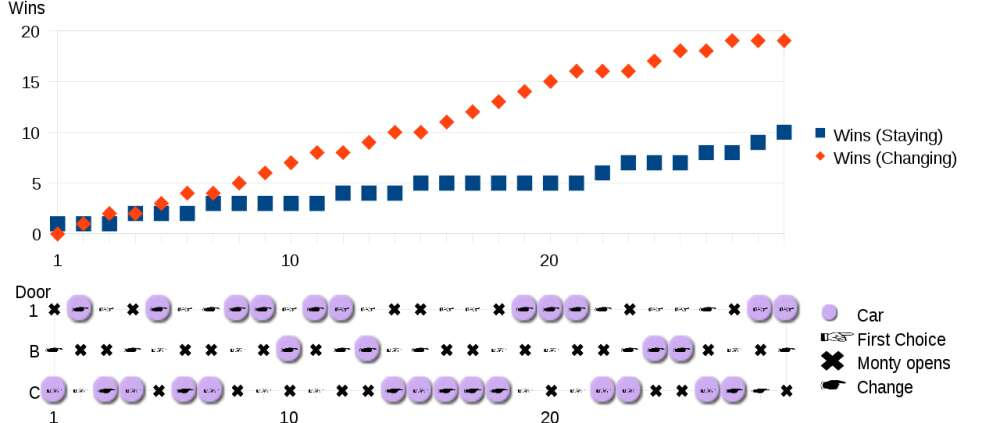It really does work - this Monte Carlo simulation of 29 rounds of the Monty Hall Problem shows that switching gets you the car about twice as often as sticking with your original choice. Image credit: Erzbischof, Wikipedia, CC BY-SA 3.0

Let’s take a look at why.

At the start of the round, you have no information. All three doors have the same likelihood of having a car behind them: 1/3.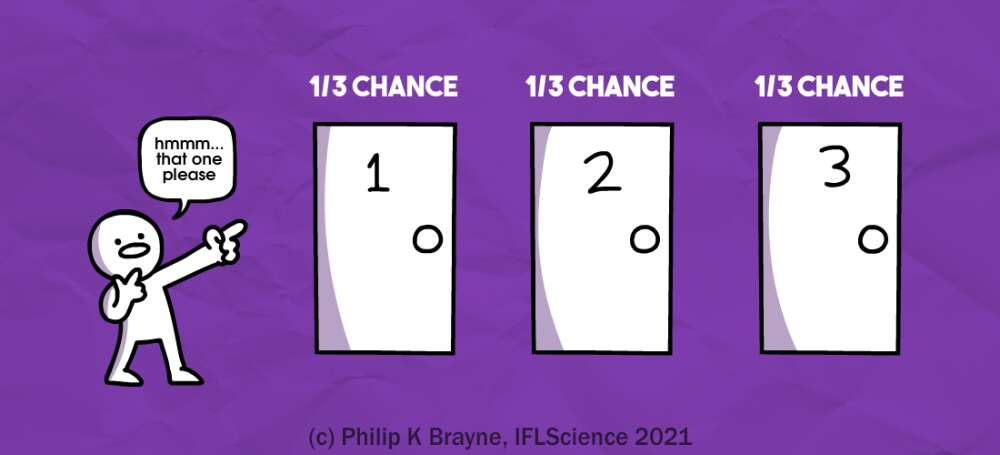So your door, door one, gives you a one in three chance of winning that car, and there’s a two in three chance that the car is behind door two or three.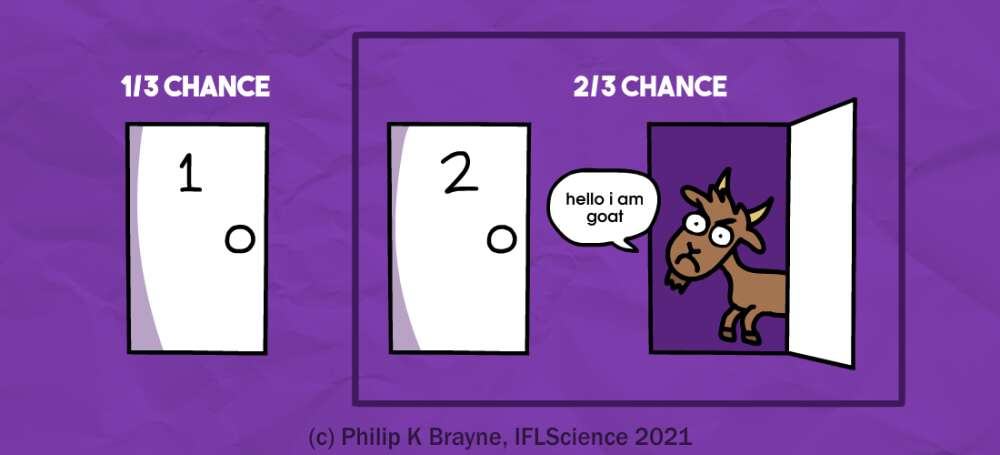But then, everything changes, because you’re given new information: the car is not behind door three. By offering you the chance to switch to door two, the gameshow host is basically offering you the choice between door one or doors two and three combined – it’s just that we now know there's a zero percent chance it’s behind door three. That leaves door two with the entire two-thirds chance of hiding a shiny new automobile.

In other words, door one gives you a one-third chance of winning, but door two gives you a two-thirds chance. By switching doors, you double your chances.

Make sense? Perhaps this will help: let’s imagine this gameshow has a million doors instead of three. Again, one of the doors has a car behind it; the other 999,999 have goats.

Again, you choose door number one, and again the host gives you new information.

“Doors three through one million have goats behind them,” he tells you. “Does that change your choice?”

So your choice now is door one, which you chose out of a million doors concealing 999,999 goats and one single car, or door two, which is the only one left after 999,998 goats have been eliminated. Thinking about it like that can make the result a bit more intuitive: there’s a one in a million chance that door one was the right pick, and so the only other door left in the gameshow must have a 999,999 in 1,000,000 chance of being the winning choice.

You don’t have to rely on your imagination to figure the problem out – cold hard math will always have your back. The reason for the unintuitive answer is something called “conditional probability”, and we can deal with that using a probability rule known as Bayes’ Theorem.

In mathematical notation, Bayes’ Theorem looks like this: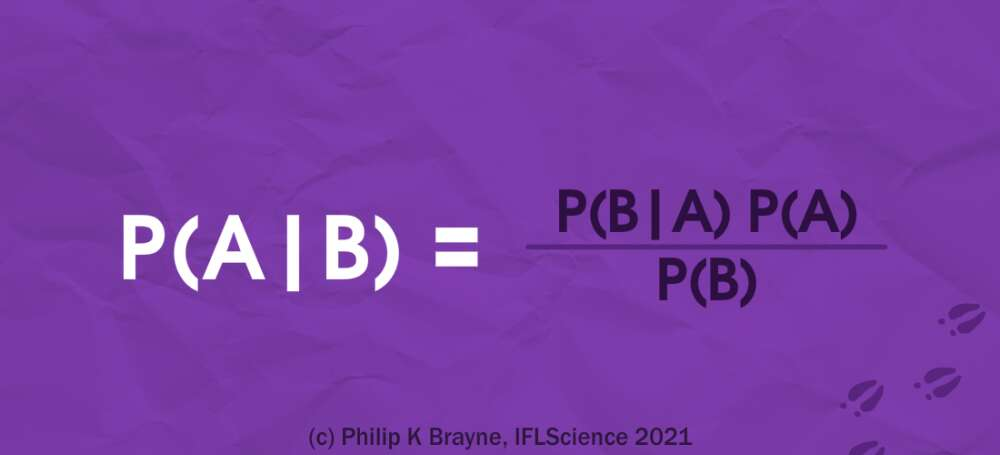In words, this says that the probability of event A occurring given that event B occurs is equal to the probability of event B occurring given that event A occurs multiplied by the overall probability of A occurring divided by the overall probability of B occurring.

OK, maybe that didn’t make it much clearer – let’s use an example. Imagine you have a bag containing three red balls and two green balls. The probability of picking out a red ball is three in five – 60 percent – and the probability of picking out a green ball is two in five, or 40 percent.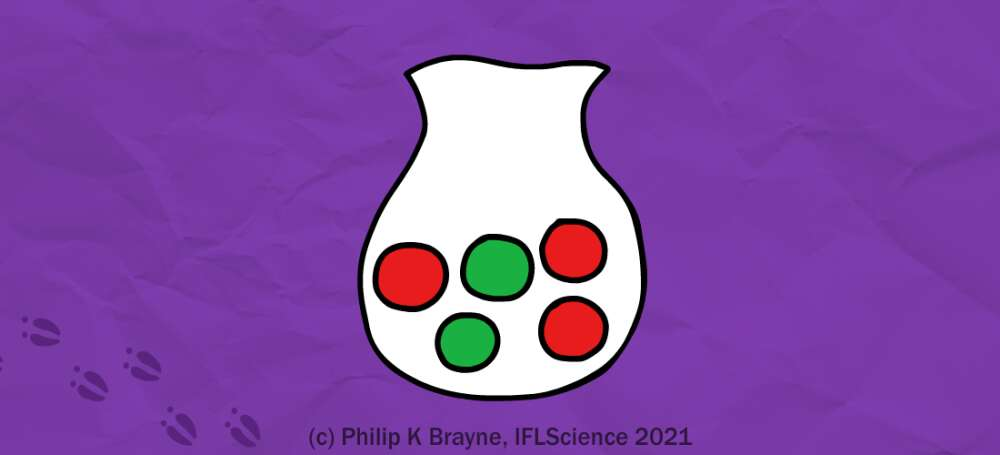But if you already picked out a red ball, those probabilities change. The number of balls is down from five to four, two red and two green, and the chance of picking either color ball is equal. On the other hand, if you already picked out a green ball, the chance of picking out another goes down to one in four, while the chance of picking a red ball becomes three-quarters.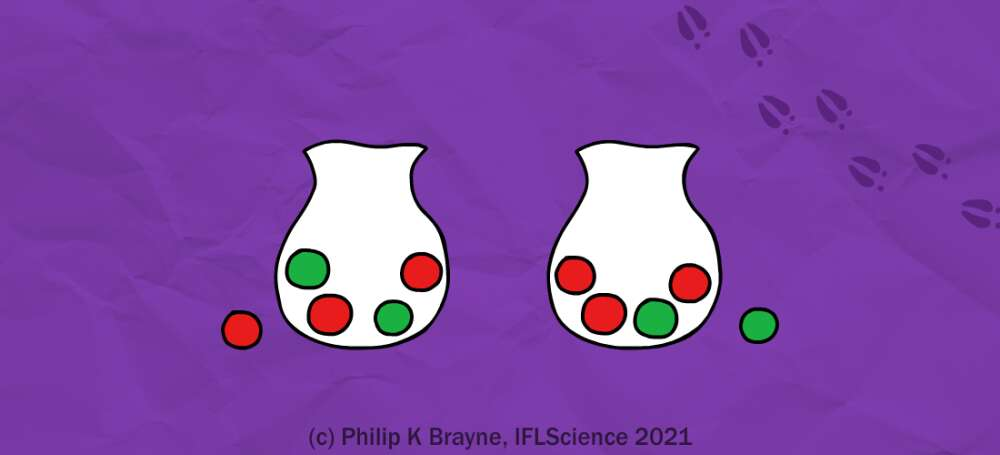Let’s apply this hypothetical to Bayes’ Theorem. We’ll call event A “picking a green ball” and event B “picking a red ball”. Then P(A|B) refers to the probability of picking a green ball given the fact that you already picked a red ball, and it’s equal to P(B|A) – the probability of picking a red ball given the fact that you already picked a green ball, which we saw was three-quarters – times P(A), the probability of picking a green ball, which is 40 percent, and divided by P(B), the probability of picking a red ball, which is 60 percent. So, Bayes' Theorem gives us a probability of one-half, just like we knew it should be.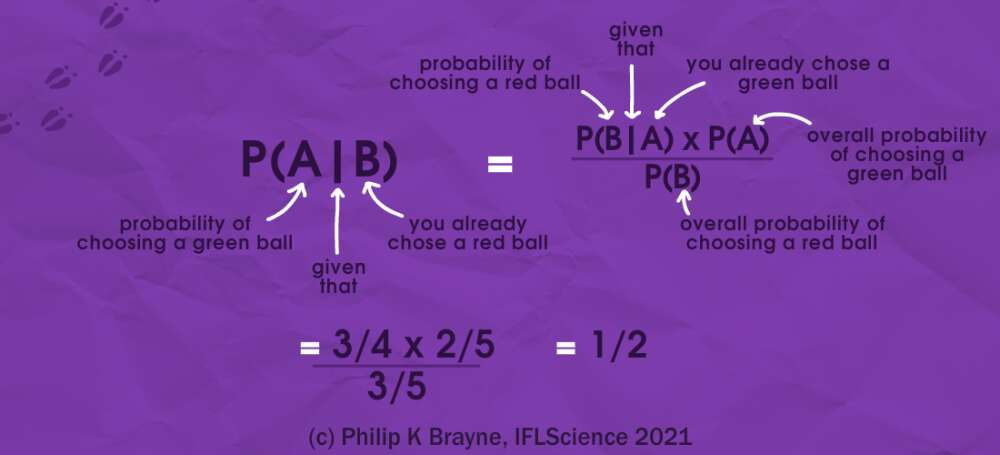So let’s use Bayes’ Theorem to solve the Monty Hall problem. We’ll call the car being behind your door “event C” (for car), and the host opening door three “event G” (for goat). Then Bayes’ Theorem looks like this: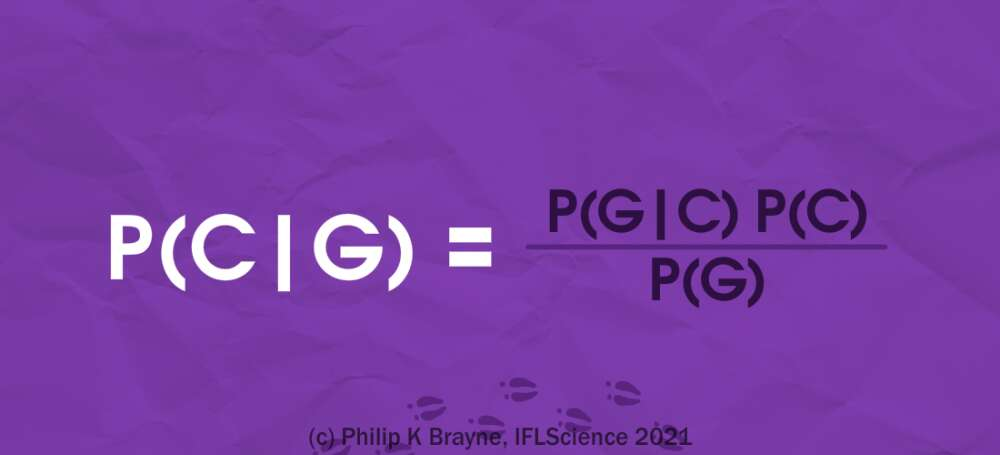Now, we know all the probabilities on the right-hand side of the equation – we just need to think a little. P(G|C) refers to the probability that the host opens door three if the car is behind door one.

Well, if the car is behind door one then both remaining doors have goats behind them, so the host can open whichever he likes – making the probability that he opens door three, one in two.

P(C) is the probability that the car is behind door one – that's one in three.

And P(G) is the probability that the host opens door three at all, and that's one-half. That's because the host will only open a door if it has a goat behind it, so he's only got a choice of two. Therefore: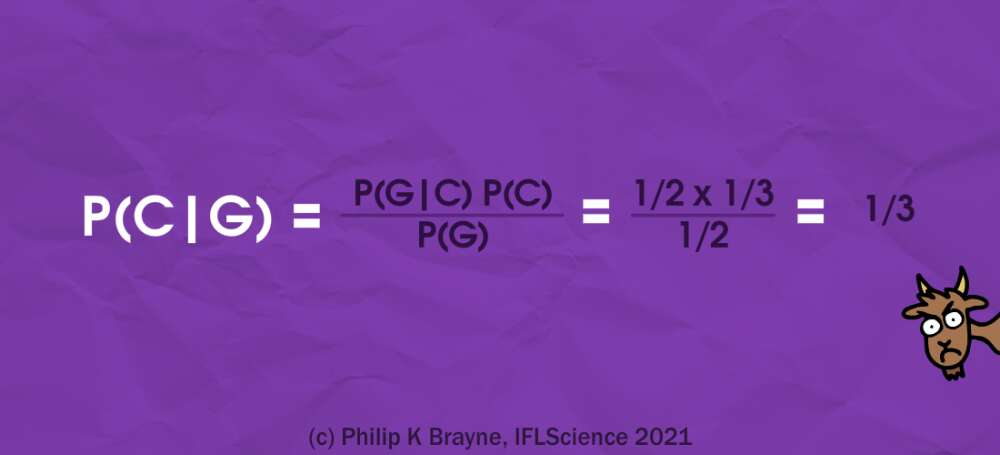The chance of the car being behind door one hasn't changed now that the host has opened door three – it's one-third. That means that the chance of the car being behind a different door (in math, we would write P(¬C) to describe that) must be two-thirds. But at this point, the only other door the car could be behind is door two!

So there you have it – the math doesn’t lie. But the Monty Hall problem isn’t the only game where conditional probability results in some perplexing results. Even more contentious is the answer to the “Boy-Girl Paradox”, or “Two Children Problem”, which says:

Mr Smith has two children. One of them is a boy. What is the probability that both are boys?

We’ll give you a minute to think it over.

If you said 50 percent, or one-half, or one in two, or anything equivalent, then [game show buzzer noise] that’s incorrect (probably). The answer is actually (probably) one in three: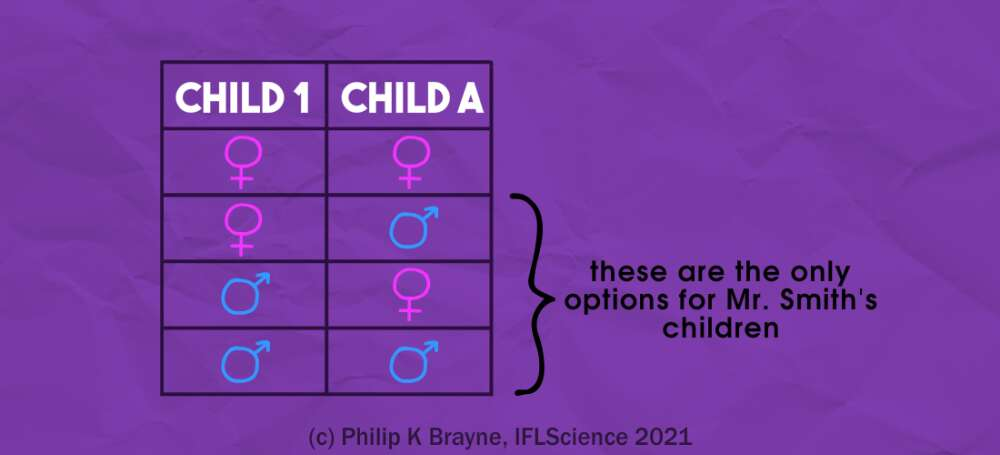Nobody who said one in two should feel bad about it though: some very clever people have made some very convincing arguments that that’s the right answer instead.

To sum up: conditional probability is a pain, and if you don’t quite understand it, you’re in good company. If it’s any consolation, the real Monty Hall – who was the host of the gameshow Let’s Make a Deal for nearly 30 years – pointed out that there’s actually a major flaw in the Problem’s setup.

“The big hole in your argument of problems is that once the first box is seen to be empty, the contestant cannot exchange his box,” he wrote in 1975 to Steve Selvin, the inventor of the Problem. “And you ever get on my show, the rules hold fast for you– no trading boxes after the selection.”

## THIS WEEK IN IFLSCIENCE

•